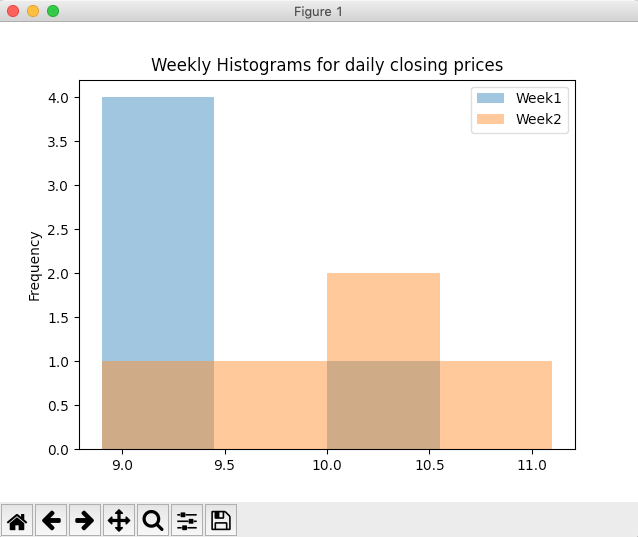# Drawing Histogram(s) For A Pandas Dataframe

## Overview:

• A histogram is a frequency plot. Each interval data is marked with different heights based on the frequency of the data in that interval. Higher the frequency greater the height marked for that frequency.
• Calling the hist() method on the plot member of a DataFrame instance draws the histogram for each column present in the DataFrame.
• Number of bins or intervals of the histogram is specified for all the columns of the DataFrame combined.
• Each histogram will be drawn in an overlapping(if any) fashion using the alpha-blending value specified.

## Example:

 # Example Python program that plots # histograms for weekly data import pandas as pds import matplotlib.pyplot as plot   # Data dailyClosingPrices = [(10.2, 9.8),  (9.4, 10.4),  (8.9, 11.1),  (9.0, 10.5),   (9.1, 9.4) ];   # The index index   = ["Mon", "Tue", "Wed", "Thu", "Fri"];   # Column labels columns = ["Week1", "Week2"];   # Create a DataFrame instance df = pds.DataFrame(data=dailyClosingPrices, index = index, columns = columns);   # Draw histograms for weekly closing prices df.plot.hist(bins=4, alpha=0.5, title="Weekly Histograms for daily closing prices"); plot.show(block=True);

## Output: